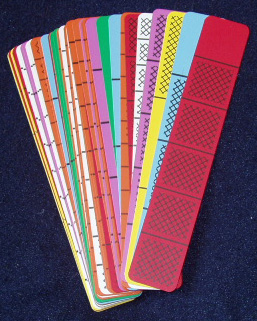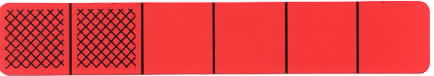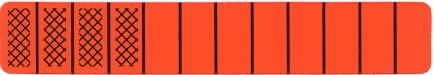Home
 Introduction to Fraction Bars Materials: Deck of Fraction Bars for each group or pair of peopleAsk each group to unbundle the decks of Fraction Bars and list or note some observations. Here a few of the many possible comments: the green bars are divided into 2 equal parts; the yellow bars are divided into 3 equal parts etc.; the bars all have the same length that is the unit is fixed; some bars are all shaded (whole bars) and some have no shading (zero bars); Discuss a full deck by asking the groups to determine the number of each type of bar. For example there are 13 different orange bars different red bars etc. There may be comments about bars with the same shaded amount such as a green bar with one part shaded and a blue bar with 2 parts shaded etc. If this type of observation does not come up ask a few questions such as: "What bars have the same amount of shading as a red bar with 2 parts shaded?"Discuss terminology and note that it is not necessary to use fractions at this introductory stage. For example "a red bar with 2 parts shaded" or " a bar with 2 parts out of 6 shaded" are sufficient to describe this bar. Note that such descriptions are nonthreatening. Even if students have been using fraction terminology such informal language for describing bars may help reduce anxiety over fractions and help them form conceptual understanding. Ask each person to select an arbitrary bar and write a description of it without using fractions. Ask a few volunteers to describe their bars. Request that the white bars (10 parts each) and the purple bars (5 parts each) be set aside as they will not be used in the following activities. Ask why the remaining types of bars form what is called "the twelfths family of fractions". (Answer: Every bar has an equal shaded amount when compared to an orange bar that is the numbers of parts in the bars (2 and 6) are all factors of 12.)